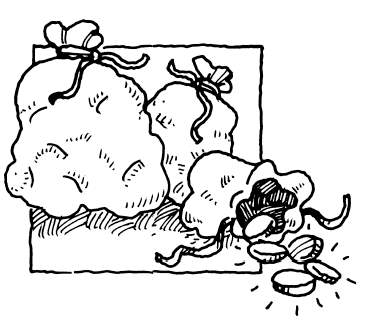### Home > PC3 > Chapter 12 > Lesson 12.2.2 > Problem12-121

12-121.Three thieves in ancient times stole three bags of gold. The gold in the heaviest bag had three times the value of the gold in the lightest bag and twice the value of the gold in the medium bag. All together, the gold amounted to $330$ florins. How much gold was in each bag? Write a system of equations and use matrices to solve the system.

$h = 3l$
$h = 2m$
$h + m + l = 330$